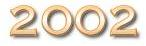Homepage Committees Other Issac Other conferences Call for papers Call for posters Call for demos Accepted papers, posters & demos Tutorials Advanced program Related events Participants Travel Information Tourist Information Registration Accommodation

[Tutorials main page] [Tutorial 1] [Tutorial 2] [Tutorial 3]

# Introduction to public key cryptography based on algorithmic number theory Pierrick Gaudry

## Motivation

In the past, cryptography was mainly used in specific and often classified areas like banking or defense. The situation has changed, especially in the last decade, since new technologies (mobile phones, Internet) required efficient, reliable but also public tools to protect the communications.

Cryptographic methods can be divided in two large classes: symmetric systems where the same key is used to cipher and to decipher, and asymmetric systems (a.k.a. public key cryptosystems) where the key used to encipher is different from the one used to decipher, the latter being impossible to deduce from the former.

Both have advantages and disadvantages. Depending on the context, one or the other will be used, and sometimes both together, mixed in complicated protocols.

Mathematical tools involved in symmetric algorithms include information theory, statistics, boolean functions. On the other hand, most of the cryptosystems used in public key cryptography rely on problems coming from number theory. The most famous, RSA, should be safe as long as we do not have efficient algorithms for factoring integers. Others rely on the difficulty of the discrete logarithm problem in a finite abelian group.

Optimizing and implementing the algorithms involved in these systems have become crucial. Some of them are highly non-trivial and make heavy use of tools coming from computer algebra or computational algebraic number theory.

## Outline

• Overview of modern cryptography

After a short history, we shall explain which are the criterion for a modern cryptosystem. The distinction between symmetric and asymmetric systems will be explained, together with their main characteristics. We shall list some tasks that can be achieved satisfactorily with today's state of the art.

• Public key cryptography based on number theory

We shall make a survey of the currently available public key cryptosystems with an emphasis on RSA and elliptic curves which are the most popular systems. For these two systems we shall give more details on the algorithmic problems that are involved and a rough idea of the state of the art.

• Example of algorithmic problem coming from cryptography: point counting on elliptic curves

As an example of a non-trivial algorithmic problem, we shall explain how the point counting problem has switched in 15 years from almost infeasible in a reasonable amount of time, to easily tackled even in a constraint environment like a PDA. This was made possible by using deep theoretical results and efficient algorithms from computer algebra.

## Target Audience

• Researchers and students in computer algebra who want to learn about this potential field of application.
• Computer scientists who want an introduction on public key cryptography.
The prerequisites for the first two parts of the presentation are very low. The third part will involve more mathematical knowledge in the area of algebraic and arithmetic geometry; we shall recall a few facts to make the talk accessible to a graduate student.

For technical remarks, contact webmaster : lemaire@lifl.fr
Last modified on Fri Jul 5 11:46:51 MET DST 2002